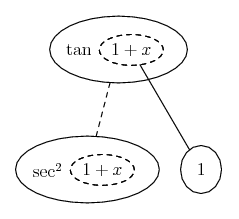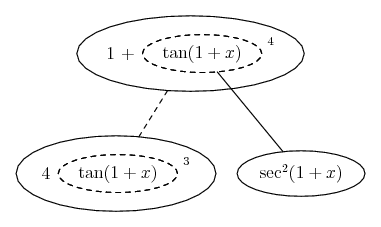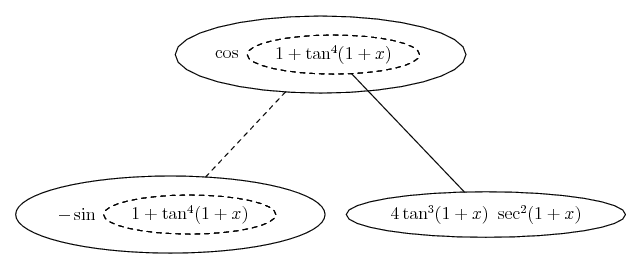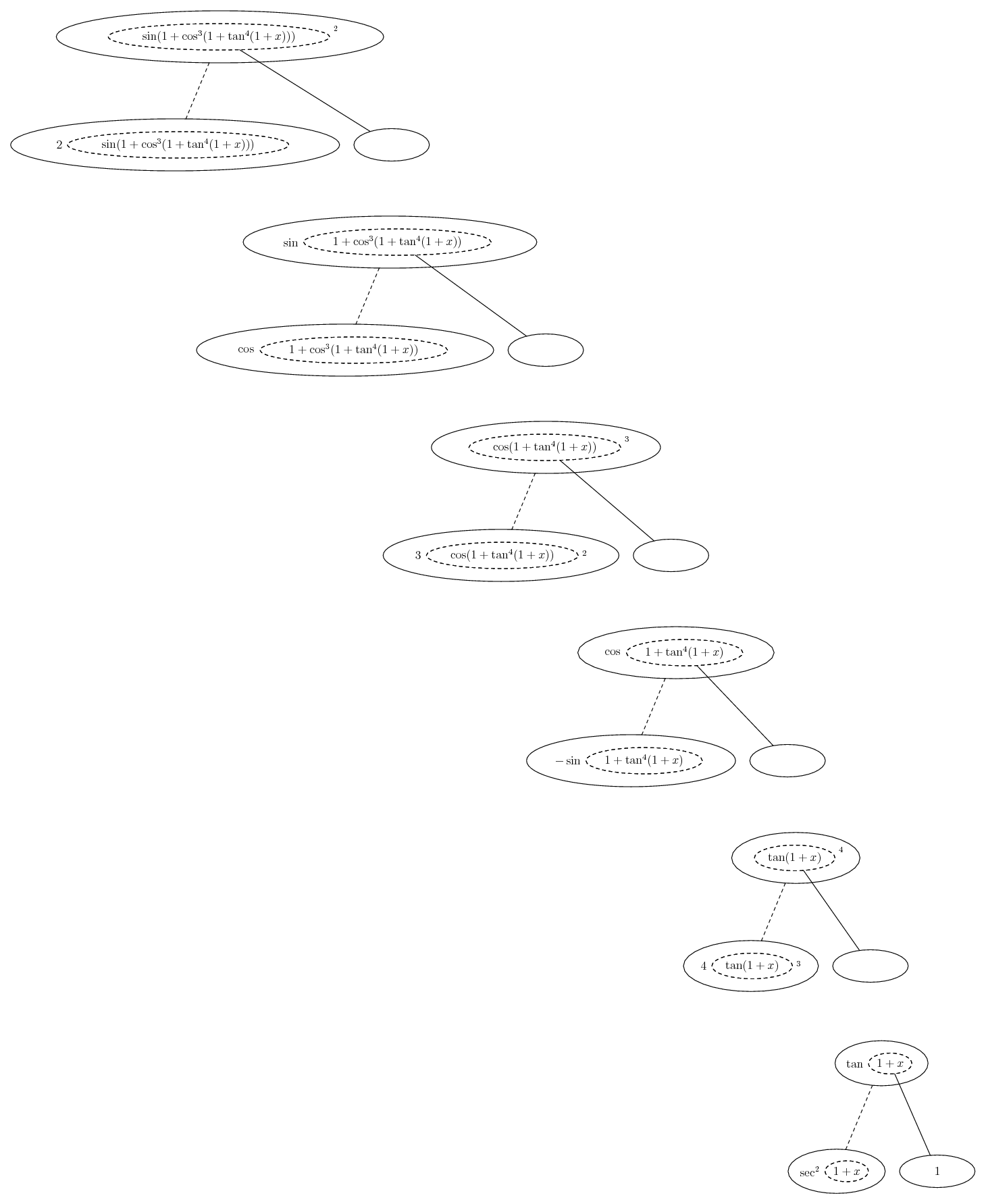# Thread: Trigonometric Derivative

1. ## Trigonometric Derivative

Hello,

I am doing a list of problems to do and have experienced some difficulty doing a particular one.

It asks to find the derivative of
f(x) = sin^2 (1+cos^3 (1+tan^4 (1+x))).

I've added a space ahead of exponents to avoid confusion, but it is all together.

Now, I don't know whether to use the chain rule multiple times, or how that would work, or anything. I don't know whether to see "(1+cos^3 (1+tan^4 (1+x)))" to sin^2 as you would see it as sin^2 x or something. I'm very confused.

Any help would be greatly appreciated, thank you!

2. By exponents do you mean brackets? If so then it might help to know you can re-write it as

f(x) = [ sin( 1 + {cos( 1 + [tan(1+x)]^4) }^3) ]^2

and yes, the chain rule nested

Here's a few more of the pieces...

$\displaystyle \frac{d}{dx}\ \tan(1+x) = \sec^2(1+x)$

... and just in case a picture helps...... so...

$\displaystyle \frac{d}{dx}\ (1 + \tan^4(1+x)) = 4 \tan^3(1+x) * \sec^2(1+x)$... so...

$\displaystyle \frac{d}{dx}\ \cos(1 + \tan^4(1+x))$

$\displaystyle = -\sin(1 + \tan^4(1+x))\ 4 \tan^3(1+x)\ \sec^2(1+x)$... so...

$\displaystyle \frac{d}{dx}\ [1 + \cos^3(1 + \tan^4(1+x))]$

$\displaystyle = 3 \cos^2(1 + \tan^4(1+x))(-\sin(1 + \tan^4(1+x)))\ 4 \tan^3(1+x)\ \sec^2(1+x)$

Etc...

Nesty nasty! Excuse myriad errors, hopefully fixed.

Or perhaps you meant exponents after all... loads of fun then!

__________________________________________

Don't integrate - balloontegrate!

Balloon Calculus Forum

Balloon Calculus Drawing with LaTeX and Asymptote!

3. Hello,

Your answer is much appreciated. Thank you very much.

Just one more simple question, now that I have the derivative
$\displaystyle \frac{d}{dx}\ [1 + \cos^3(1 + \tan^4(1+x))]$

should I continue to work at my answer such that I use the product rule to find

$\displaystyle \frac{d}{dx}\ sin^2$ * $\displaystyle [1 + \cos^3(1 + \tan^4(1+x))]$ + $\displaystyle \frac{d}{dx}\ [1 + \cos^3(1 + \tan^4(1+x))]$ * $\displaystyle sin^2$ ?

4.Originally Posted by Torcida1911Just one more simple question,
Unless I'm missing a trick*, there's no way to keep it simple! This problem is unusually messy, but I guess the idea is that by the end you know the chain rule...

Not product rule - remember sin^2(x) means (sin(x))^2

There are 2 more layers to deal with: sin and then squaring.

$\displaystyle \frac{d}{dx}\ \sin[1 + \cos^3(1 + \tan^4(1+x))]$

will be cos of the same square-bracket-ed expression and then that multiplied by the derivative of same square-bracket-ed expression - which is the last result we got.

For what it's worth, I'll do a pic working from the outside in...

*We could maybe use some identities before starting to differentiate... not sure... don't think so

OK, here's the big pic, in a 'spoiler' - on this occasion so we don't spoil the page layout rather than the fun. The whole derivative should be the product of all the left forks. You can simplify that result, a bit.

Spoiler:5. I've got it.. what an intense problem..

Thank you so much for your time and effort. Great visuals.

#### Search Tags

derivative, trigonometric